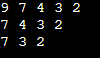# Deleting a Node from a Circular Linked List in C

## Deleting a Node from a Circular Linked List in C Language

In this article, I am going to discuss Deleting a Node from a Circular Linked List in C Language with Examples. Please read our previous article, where we discussed Inserting a Node in a Circular Linked List in C Language with Examples. At the end of this article, you will understand how to delete a node in a Circular Linked List with algorithms and examples using C Language.

##### Deleting From Circular Linked List using C Language:

There are two cases for deletion just similar to a linear linked list.

2. Deleting a Node from a Given Position in a Circular Linked List.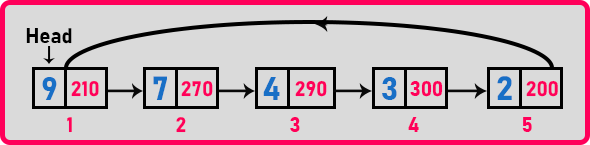If we delete the head node or the first node then we have to make another node as a head node in the circular linked list. So we will move head. We can select any node as a head node because it is a circular linked list. So we will be selecting just the next node as a head node.

The next method is deleting a node from the given position. We can delete any node i.e. 1st, 2nd, or 5th node, or delete a node from any given position. This procedure is the same as deleting from the linear linked list. So, first, let us look at the second method.

##### Deleting A Node from the Given Position using C Language:

Let us delete a node from the 4th index of the linked list.So we want to delete the ‘3’ node. If we want to delete the 4th node then we have to manipulate the node before the 4th node i.e. 3rd node. And make the 3rd node point on the 5th node. The only we can delete is the 4th node. To access the 3rd and 4th nodes, we need a pointer on the 4th node as well as on the 3rd node (tail pointer). One pointer will be moving and the second pointer will be following the pointer. So we can bring two pointers from the beginning of the linked list or we can bring a pointer to 3rd node and take one more pointer on the next node. So two options are there.

Now we will bring just one pointer from the head to 3rd node then we take one more pointer on the next node. So we will be moving just one pointer. Let us take a pointer ‘p’ on the head node,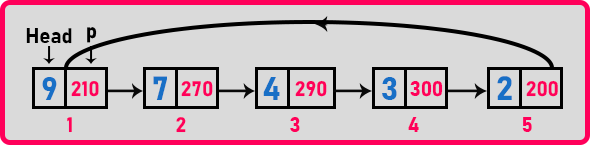We want to delete the 4th node, so we should bring p on the 3rd node.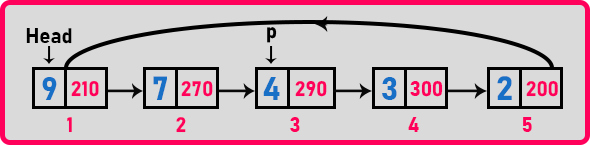We have moved just 2 steps. 4th nodes means 2 steps, 5th nodes means 3 steps. So for deleting any node we have to move n – 2 steps. So the pseudo-code for this step is,

for (i = 0; i < pos-2; i++){
p = p->next;
}

Now we need a pointer on the 4th node also. So take another pointer ‘q’ and assign it to p->next,

q = p->next;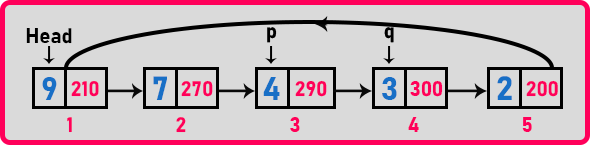Here, q is pointing to p’s next node. Now, what changes do we have to make? P’s next should point on q’s next.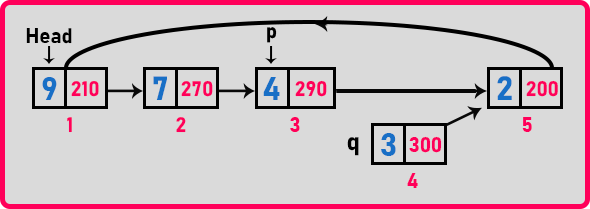Now both p’s next and q’s next pointing on the 5th node. Now we can easily delete the 4th node because it is not the part of linked list. No node is pointing to the 4th node. We will take out the value of the 4th node and then delete the node.

int x = q->data;
delete q;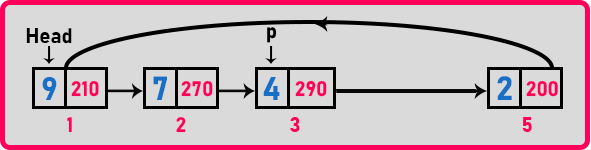So we have deleted the 4th node from the linked list. This procedure is the same as deleting from the linear linked list. The pseudo-code for this procedure is given below,

for (i = 0; i < pos-2; i++){
p = p->next;
}
q = p->next;
p->next = q->next;
int x = q->data;
delete q;

Now let us see deleting the head node.

##### Deleting Head Node from a Circular Linked List using C Language:

If we are deleting the head node, we can make the next node as the head node but the last node should point to the new head node. So we have to do this modification to the new head node.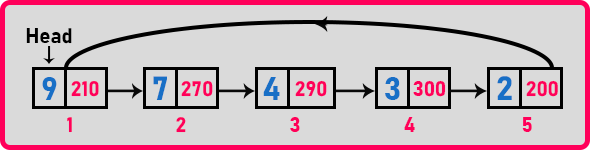Now for deleting the head node, we should take a pointer p on the head node and move the p pointer until it reaches the last node as per the above head node,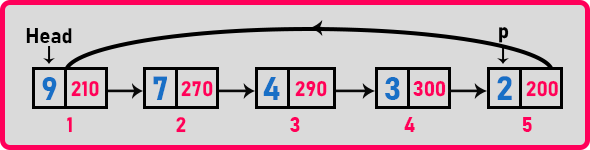Now we have to make p’s next to point on the head’s next node.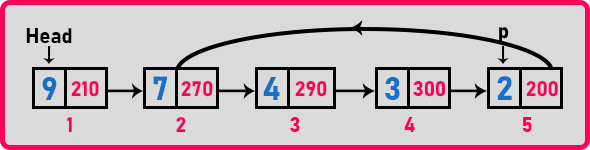Here links have changed and now we have to make p’s next as Head. For that, first, we have to delete the head node,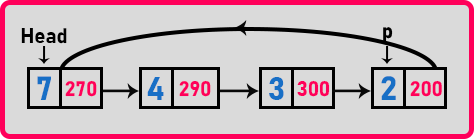Here, we have deleted the head’s node and made the p’s next as the head. So these are the steps for deleting the head node from the circular linked list. The pseudo-code for this procedure is given as,

p = p->next;

##### Time Complexity:

The time taken for deleting the head node is n because we have to take p to the last node of the linked list. And the time taken for deleting any given node, the minimum is constant, and the maximum is n. If we are deleting 2nd node then the time will be constant otherwise it will be n. Now let us look at the complete program.

##### Example to understand the deletion process in Circular Linked List using C Language:
```#include <stdio.h>
#include <stdlib.h>
struct Node {
int data;
struct Node * next;

void create(int A[], int n) {
int i;
struct Node * t, * last;
Head = (struct Node * ) malloc(sizeof(struct Node));

for (i = 1; i < n; i++) {
t = (struct Node * ) malloc(sizeof(struct Node));
t -> data = A[i];
t -> next = last -> next;
last -> next = t;
last = t;
}
}

void Display(struct Node * h) {
do {
printf("%d ", h -> data);
h = h -> next;

printf("\n");
}

void RDisplay(struct Node * h) {
static int flag = 0;

if (h != Head || flag == 0) {
flag = 1;
printf("%d ", h -> data);
RDisplay(h -> next);
}

flag = 0;
}

int Length(struct Node * p) {
int len = 0;
do {
len++;
p = p -> next;

return len;
}

void Insert(struct Node * p, int index, int x) {
struct Node * t;
int i;

if (index < 0 || index > Length(p))
return;

if (index == 0) {
t = (struct Node * ) malloc(sizeof(struct Node));
t -> data = x;
} else {
while (p -> next != Head) p = p -> next;
p -> next = t;
}

} else {
for (i = 0; i < index - 1; i++) p = p -> next;
t = (struct Node * ) malloc(sizeof(struct Node));
t -> data = x;
t -> next = p -> next;
p -> next = t;
}
}

int Delete(struct Node * p, int index) {
struct Node * q;
int i, x;

if (index < 0 || index > Length(Head))
return -1;
if (index == 1) {
while (p -> next != Head) p = p -> next;
} else {
p -> next = Head -> next;
}
} else {
for (i = 0; i < index - 2; i++)
p = p -> next;
q = p -> next;
p -> next = q -> next;
x = q -> data;
free(q);
}
return x;
}

int main() {
int A[] = {9, 7, 4, 3, 2};
create(A, 5);